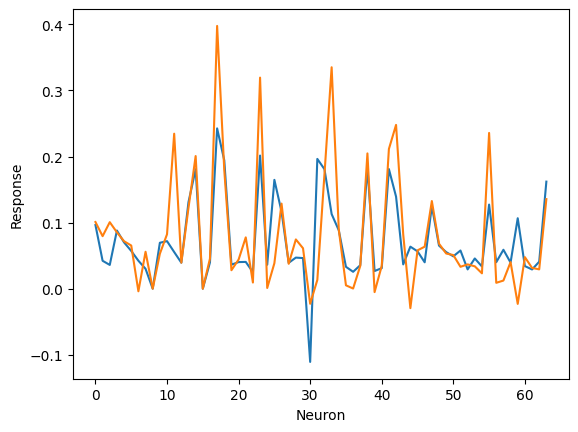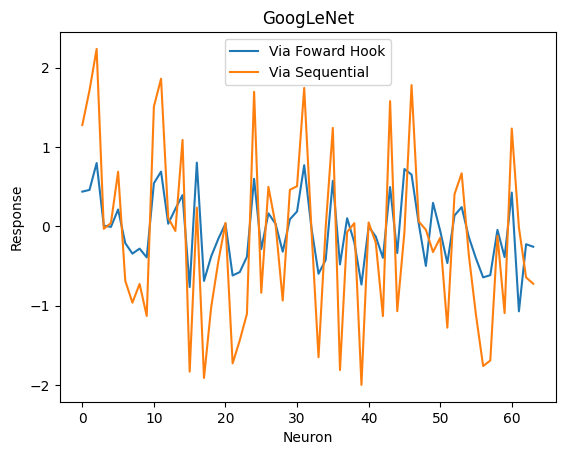# Confusion with BatchNorm2D calculation and running a subset of layers

Hello,

I want to better understand the BatchNorm2D layer, so I am attempting to do a manual calculation but am unable to reproduce the results of BatchNorm2D. I have two issues: the first is with my understanding BatchNorm2D itself and the second is with regards to only running a subset of layers.

Problem 1: BatchNorm2D calculation
I am starting with the pretrained DenseNet121 and passing in a uniform image. Then I take the outputs of the 1st Conv2D layer and the 1st BatchNorm2D layer. I then take the statistics of the BatchNorm2D layer and try to use them to transform the outputs of Conv2D to get the same output.

``````mod = models.googlenet(pretrained=True)

#Generate a stimulus
stim = np.ones([224,244,3])
stims_t = np.moveaxis(stim[np.newaxis], -1, 1)
stims_t = torch.tensor(stims_t)

#Get statistics from the first BatchNorm2D
running_mean = mod.conv1.bn.running_mean.detach().numpy()
running_var = mod.conv1.bn.running_var.detach().numpy()
eps = mod.conv1.bn.eps
gamma = mod.conv1.bn.weight.detach().numpy()
beta = mod.conv1.bn.bias.detach().numpy()

#Get the output from just the first Conv2D
cnn = nn.Sequential(mod.conv1.conv)
out = cnn(stims_t.float())
out = out.detach().numpy().squeeze()
center = int(np.shape(out)[-1]/2) #I only want the output from the center convolution
conv1 = out[:,center,center]

#Get the output from the first BatchNorm2D
cnn2 = nn.Sequential(mod.conv1.conv, mod.conv1.bn)
out = cnn2(stims_t.float())
out = out.detach().numpy().squeeze()
center = int(np.shape(out)[-1]/2)
conv1_2 = out[:,center,center]

### Manual calculation ###
#(Attempt to) Transform Conv2D outputs into BatchNorm2D outputs
faux_conv1_2 = (conv1 - running_mean) / np.sqrt(running_var + eps)*gamma + beta

#Compare the true and faux normalizations:
plt.figure()
plt.plot(conv1_2)
plt.plot(faux_conv1_2)
plt.xlabel('Neuron')
plt.ylabel('Response')
``````I would really appreciate anyone being able to tell me what is wrong with my calculation.

From my understanding of the documentation, any momentum calculations aren’t used during evaluation, and affine is set to True for DenseNet121, so I should be including gamma and beta.

Problem 2: Only running subset of layers via nn.Sequential
My second problem is related to the method I used to only run the first layers of DenseNet, wherein I took out the first few layers and put them back together with nn.Sequential. This method seems to work fine for DenseNet121 and AlexNet but fails for GoogLeNet when compared to using a forward hook. The main purpose of doing it this way to is to get the responses of intermediate layers while also not running the whole network. Is there something inherently wrong with my approach or am I missing something important about GoogLeNet?

``````#Define the hook
def hook(module,inp,output):
center = int(output.shape[-1]/2)
r_center = output[..., center, center]#just store responses from center of feature map
outputs.append(r_center)

#Generate a stimulus
stim = np.ones([224,244,3])
stims_t = np.moveaxis(stim[np.newaxis], -1, 1)
stims_t = torch.tensor(stims_t)

#For GoogLeNet (same method used in DenseNet and AlexNet)

for modl in mod.children(): #loop through every module
for layer in modl.children(): #loop through every layer in the module
if isinstance(layer, nn.Conv2d): #if it is a conv layer
layer.register_forward_hook(hook)
elif isinstance(layer, nn.ReLU):
layer.inplace = False #set inplace rectification to False to get unrect responses

mod2 = nn.Sequential(mod.conv1.conv) #replace with mod.features.conv0 for DenseNet121 or mod.features for AlexNet

#Get response from forward hook method
outputs = []
r = mod(stims_t.float())
conv1 = outputs
conv1 = conv1.detach().numpy().squeeze()

#Get response from Sequential method
out = mod2(stims_t.float())
out = out.detach().numpy().squeeze()
center = int(np.shape(out)[-1]/2)
conv1_2 = out[:,center,center]

print(conv1)
print(conv1_2)
``````

The response of the first neuron via the forward hook is 0.43627724 while the response with nn.Sequential is 1.275382.

For the first issue: check my manual batchnorm implementation to see how the layer is working internally.
For the second issue: re-wrapping models into `nn.Sequential` containers can easily break them e.g. if functional calls were used in the original `forward` method. In `GoogleNet` a new functional calls are used such as the conditions and flattening operation so you might want to check if this might be causing the issue.

Thank you for your rapid response! The manual batchnorm implementation is very helpful and this gives me something to keep working on.

As for the second problem, I should only be calling the very first conv layer of the first BasicConv2d module, which in of itself only solely consist of conv2d, batchnorm2d and a ReLU function. So I’m still not sure why it’s different than when using a forward hook.

That being said, what I ultimately care about is getting the outputs from certain intermediate layers and then not proceeding further. How would you recommend I accomplish this goal without nn.Sequential?

Note that the relu activations are applied inplace in the original model as seen here. The forward hook would store the output of the corresponding batchnorm layer, but if you do not `.clone()` it the next `F.relu(x, inplace=True)` op would change it.
Could you check if this could be the case?

So in the original code I posted comparing the hook vs nn.Sequential, I only looked at the first conv layer and no further so as not to include batchnorm nor the relu operation. Yet, I still saw differences.However, I did advance the hook to the first batchnorm (I left relu inplace) and advanced nn.Sequential to as well and then they matched pretty closely but with the hook method being obviously relu’ed (not shown).